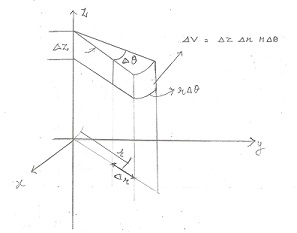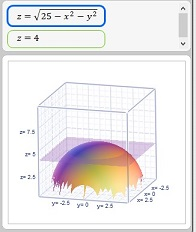# Set up the integral to calculate the volume in cylindrical coordinates bounded by z=...

## Question:

Set up the integral to calculate the volume in cylindrical coordinates bounded by

{eq}z= \sqrt{25-r^2}, \ z=4, \ r=4 {/eq}.

## Cylindrical Coordinates, Volume of a Solid:

The volume of a solid region T, is given by the triple integral,

{eq}\displaystyle \iiint_T \; dV. {/eq}

When the solid is cylindrical in shape, we resort to cylindrical coordinates, which are a combination of the polar coordinates, and the z - coordinate. The cartesian coordinates are represented as,

{eq}x = r \cos \theta \\ y = r \sin \theta \\ z = z {/eq}

The differential volume element in cylindrical coordinates becomes,

{eq}dV = dz \; r \; dr \; d \theta {/eq}.

The volume expression is of a small solid shape like the bite off of a pizza slice.

The above concept can be assimilated from the following figure:Become a Study.com member to unlock this answer! Create your account

Observe the graph of the solid region bounded by {eq}z= \sqrt{25 - r^2}, \ z=4 {/eq}.The region can be parametrized using cylindrical...Cylindrical & Spherical Coordinates: Definition, Equations & Examples

from

Chapter 13 / Lesson 10
19K

In this lesson, we introduce two coordinate systems that are useful alternatives to Cartesian coordinates in three dimensions. Both cylindrical and spherical coordinates use angles to specify the locations of points, a feature they share with 2-D polar coordinates.